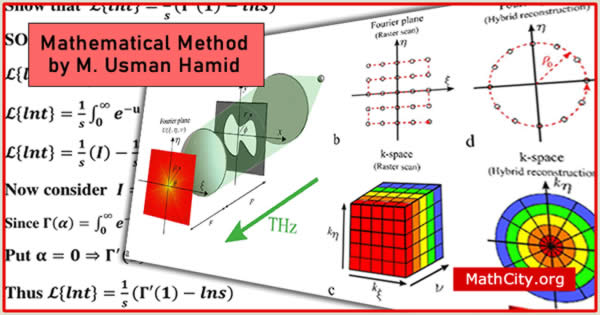# Mathematical Method by Muhammad Usman HamidThese notes are send by Muhammad Usman Hamid. We acknowledged his efforts to published these notes on MathCity.org.

The main objective of this course is to provide the students with a range of mathematical methods that are essential to the solution of advanced problems encountered in the fields of applied physics and engineering. In addition this course is intended to prepare the students with mathematical tools and techniques that are required in advanced courses offered in the applied physics and engineering programs.

• Name: Mathematical Method
• Provider: Mr. Muhammad Usman Hamid
• Pages: 179 pages
• Format: PDF
• Size: 3.76 MB

Fourier Methods: The Fourier transforms. Fourier analysis of the generalized functions. The Laplace transforms. Hankel transforms for the solution of PDEs and their application to boundary value problems.

Green’s Functions and Transform Methods: Expansion for Green’s functions. Transform methods. Closed form Green’s functions.

Variational Methods: Euler-Lagrange equations. Integrand involving one, two, three and n variables. Special cases of Euler-Lagrange’s equations. Necessary conditions for existence of an extremum of a functional. Constrained maxima and minima.

Perturbation Techniques: Perturbation methods for algebraic equations. Perturbation methods for differential equations.

• notes/mathematical-method-usman-hamid